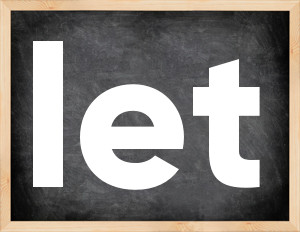# Let past tenseThe English verb 'let' is pronounced as [lɛt].
Related to: irregular verbs.
3 forms of verb let: Infinitive (let), Past Simple - (let), Past Participle - (let).

## Here are the past tense forms of the verb let

👉 Forms of verb let in future and past simple and past participle.
❓ What is the past tense of let.

## Let: Past, Present, and Participle Forms

Base Form Past Simple Past Participle
let [lɛt]

let [lɛt]

let [lɛt]

## What are the 2nd and 3rd forms of the verb let?

🎓 What are the past simple, future simple, present perfect, past perfect, and future perfect forms of the base form (infinitive) 'let'?

### Learn the three forms of the English verb 'let'

• the first form (V1) is 'let' used in present simple and future simple tenses.
• the second form (V2) is 'let' used in past simple tense.
• the third form (V3) is 'let' used in present perfect and past perfect tenses.

## What are the past tense and past participle of let?

The past tense and past participle of let are: let in past simple is let, and past participle is let.

### What is the past tense of let?

The past tense of the verb "let" is "let", and the past participle is "let".

### Verb Tenses

Past simple — let in past simple let (V2).
Future simple — let in future simple is let (will + V1).
Present Perfect — let in present perfect tense is let (have/has + V3).
Past Perfect — let in past perfect tense is let (had + V3).

### let regular or irregular verb?

👉 Is 'let' a regular or irregular verb? The verb 'let' is irregular verb.

## Examples of Verb let in Sentences

•   Maily, just let him run (Present Simple)
•   I will not let her lose \$29000 (Future Simple)
•   It seems our uncle has let us down once again (Present Perfect)
•   I feel Paul has let go of most of his anger (Present Perfect)
•   If the authorities had let him finish... (Past Perfect)
•   Don't let me down and you will be safe (Present Simple)
•   My parents never let me buy clothes on my own (Present Simple)
•   If he lets her go, it will be a huge tragedy (Present Simple)
•   Today we are letting our animals go (Present Continuous)
•   Tell me the truth and I will let you call your family (Future Simple)

Along with let, words are popular jump and must.

Verbs by letter: , , , , , , , , , , , , , , , , , , , , , , , , .